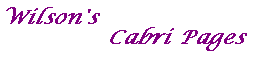#### Proof of the Dual Conic Theorem

Some Definitions
A hexagon has the Pascal Property if the intersections of opposite sides are collinear.
A hexagon has the Brianchon Property if the diagonals (i.e. lines joining opposite vertices) are concurrent.

Pascal's Theorem states that a hexagon inscribed in a conic has Pascal's Property.
The Converse of Pascal's Theorem states that the vertices of a hexagon with Pascal's Property lie on a conic.
Brianchon's Theorem states that a hexagon circumscribed round a conic has Brianchon's Property.

The Dual Conic Theorem

Suppose that C and D are conics.
Then the dual of D with respect to C is a conic.

Proof
Throughout the proof duality means duality with respect to C.

Let A,B,C,D and E be distinct points on D, fixed once for all.
Then D is the unique conic determined by A .. E.

Let F be any other point on D, and
let H be the hexagon composed of the tangents to D at these six points.

By Brianchon's Theorem, the hexagon H has the Brianchon Property,
and so the dual hexagon H' has the Pascal Property.

Thus, by the Converse of Pascal's Theorem,
the vertices of H' lie on a conic E.

The vertices of H' correspond to A .. F (being the duals of the tangents to D at these points).
Since A .. E are fixed, so is the conic throught the vertices of H'.

As F varies on D, the locus of the dual of the tangent at F will be the conic E.